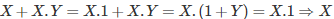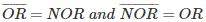# MCQs on Digital Electronics

##### Page 4 of 35. Go to page 1 2 3 4 5 6 7 8 9 10 11 12 13 14 15 16 17 18 19 20 21 22 23 24 25 26 27 28 29 30 31 32 33 34 35
01․ In the boolean algebra, a variable has ________ different state(s)/value(s).
1
2
3
4

Boolean Algebra is based on binary number system which has two different states either 1 or 0.

02․ In Boolean algebra A.A is equal to
A
2A
1

In Boolean algebra, A.A = A, where A is a variable having value either 1 or 0.

03․ AND operation is equivalant to
Division
Union
Intersection
Both B and C

Logical AND operation means Y = A.B i.e. A AND B = A Intersection B. It results in 1, if and only if all the inputs are 1.

04․ OR operation is equivalant to
Division
Union
Intersection
Both B and C

Logical OR operation means A OR B = A Union B (Y = A + B). It results in 1, if at least one input is 1.

05․ In Boolean algebra AND is represented by
+
-
/
×

In Boolean algebra, logical AND is represented by ×, A AND B = A × B.

06․ In Boolean algebra, OR is represented by
+
-
/
×

In Boolean algebra logical OR is represented by +, A OR B = A + B.

07․ X + X.Y = ?
1
0
X
Y

By applying Absorption property in Boolean algebra,.

08․ LSI means
Large Symbol Integration
Large Scale Integration
Large Symbol Invertor
Large Scale Invertor

LSI means Large Scale Integration. The abstraction level of the Integrated circuits are characterized as
• Small Scale Integration
• Medium Scale Integration
• Large Scale Integration
• Very Large Scale Integration

09․ Truth table is used to express
Boolean expression
Boolean map
Boolean matrix
OR is the complement of NOR and reverse logic is also true..